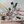Bad Circuits wrote 10/13/2020 at 08:15 • 2 min read • Like

The circuit below is a bad ultrasonic biasing design:

In the circuit the ultrasonic sensor is modelled with infinite output resistance. In practice, the resistance could be no more than about 1 kohm. However, this resistance has almost nothing to do with the concept that I am trying to explain in this article.

If the DC voltage across the sensor is 0.1 V, then the DC current entering the sensor will be about (ignoring the Rl resistor) 2.9 V / 1 kohm = 2.9 mA. Although ultrasonic sensors are dissipate high AC currents, it is my opinion that such a high DC current can damage the ultrasonic sensor. Ultrasonic sensor is made with thin contact wires so that they can vibrate at frequencies as high as 40 kHz.

Also, ultrasonic sensor can be modelled as a resonant RLC circuit. There could be a parallel inductive component across the sensor terminals. This component is short circuit in DC steady state that will drain high current from power supply if the Rs resistor is 10 ohms. We all know that electrical power relays are also inductors and this is why those relays need potection circuits to protect the semiconductor drivers. Because the ultrasonic coil of a typical ultrasonic sensor is so small in inductance, such protection circuit are not needed.

The correct solution is to completely remove the Rs resistor (replace the Rs resistor with an open circuit). There are circuits on the internet that are published with a 100 kohm resistor Rs resistor value. The design of those circuits could be correct.

## Discussions